Disclaimer: This is an example of a student written essay.

Any scientific information contained within this essay should not be treated as fact, this content is to be used for educational purposes only and may contain factual inaccuracies or be out of date.

# Evolutionary Polynomial Regression

 ✅ Paper Type: Free Essay ✅ Subject: Engineering ✅ Wordcount: 1501 words ✅ Published: 31st Aug 2017

Evolutionary polynomial regression (EPR) is a data mining technique based on evolutionary computing that was developed by Giustolisi and Savic (2006). It combines the power of genetic algorithm with numerical regression to develop symbolic models. EPR is a two-step technique in which, at the first step, exponents of symbolic structures are searched using a genetic algorithm (GA) that is the key idea behind the EPR, and in the second step, the parameters of the symbolic structures are determined by solving a linear least squares problem.  The general symbolic expression used in EPR can be presented as follows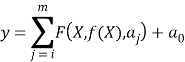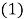Where y is the estimated output of the process, m is the total number of the polynomial terms which excludes the bias term a0, F is a function constructed by the process, X is the matrix of independent input variables, f is a function defined by the user, and aj is a constant value for jth term.

The first step and key idea in identification of the model structure in EPR is to transfer Equation 1 into the following vector form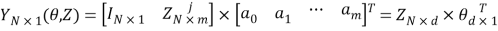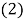Where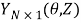is the least-squares estimate vector of the N target values;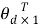is the vector of d=m+1 parameters aj and a0 (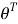is the transposed vector); and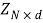is a matrix formed by(unitary vector) for bias a0, and m vectors of variables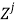. For a fixed j, the variablesare a product of the independent predictor vectors of inputs,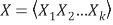.

EPR starts from Equation 2 and searches for the best structure, i.e. a combination of vectors of independent variables (inputs)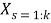.

The matrix of input X is given as :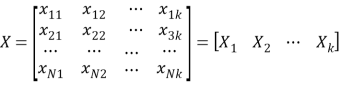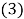Where the kth column of X represents the candidate variable for the j th term of Equation 2. Therefore the jthterm of Equation 2 can be written as: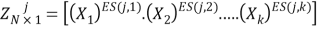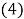Where, Z jis the jthcolumn vector in which its elements are products of candidate independent inputs and ES is a matrix of exponents. Therefore, the problem is to find the matrix ESkmof exponents whose bounds are specified by the user. For example, if a vector of candidate exponents for inputs, X , (chosen by user) is EX=[0,1,2] and number of terms (m) (excluding bias) is 4, and the number of independent variables (k) is 3, then the polynomial regression problem is to find a matrix of exponents ES 4-3 . An example of such a matrix is given here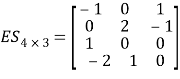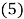Each exponent in ES corresponds to a value from the user-defined vector EX. Also, each row of ES determines the exponents of the candidate variables of jth term in equations (2). By implementing the above values in equation (4), the following set of expressions is obtained: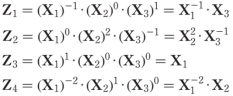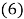Therefore, based on the matrix given in equation (5), the expression of equation (2) is given as: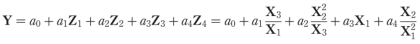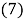In the next stage, the adjustable parameters, aj, can now be computed, by means of the linear Least Squares (LS) method.

The original EPR methodology was based on Single-objective Genetic Algorithm (SOGA) for explore the space of solutions while penalizing complex model structures using some penalization strategies.

In this method, in the first stage, the maximum value for the number of terms (m) is assumed then a consecutive search for the formulas having 1 to m terms is undertaken. To accelerate convergence, the results obtained in each stage of search could be randomly entered into the population of the next stage search .

However the single-objective EPR methodology showed some drawbacks, and therefore the multi-objective genetic algorithm (MOGA) strategy has been added to EPR.

In 2006, Guistolisi and Savic (2006) improved the EPR technique to overcome these shortcomings, using Multi-Objective Generic Algorithm (MOGA) instead of SOGA. The main features of the developed method are as follows :

1) Increasing the model accuracy,

2) Reducing the number of polynomial coefficients,

3) Minimization of the number of inputs (e.g. the number of times each Xi appears in the model).

In the developed version, a simultaneous search is conducted for polynomials having 1 to m coefficients; consequently, it is faster than the previous version (i.e., SOGA).

In order to determine all models corresponding to the optimal trade-off between structural complexity and fitness level of the model, The EPR technique is Equipped with a range of objective functions which help to optimize the result based on Pareto dominance criterion.

The objective functions used are: (i) Maximization of the fitness; (ii) Minimization of the total number of inputs selected by the modeling strategy; (iii) Minimization of the length of the model expression.

The objective functions mentioned above can be used in a two objective configuration or all together. In which one of them will limit the complexity of the models, while at least one objective function controls the fitness of the models.

In this study the multi-objective EPR is used to develop the EPR-based models.

The coefficient of determination (COD) which is used to evaluated the level of models accuracy at each stage is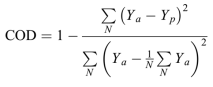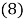Where Ya is the actual measured output value; Yp is the EPR-predicted value, and N is the number of data points in which the COD is computed.

View all

## DMCA / Removal Request

If you are the original writer of this essay and no longer wish to have your work published on UKEssays.com then please:

Related Services

Prices from

QAR553

Approximate costs for:

• 1000 words
• 7 day delivery

Related Lectures